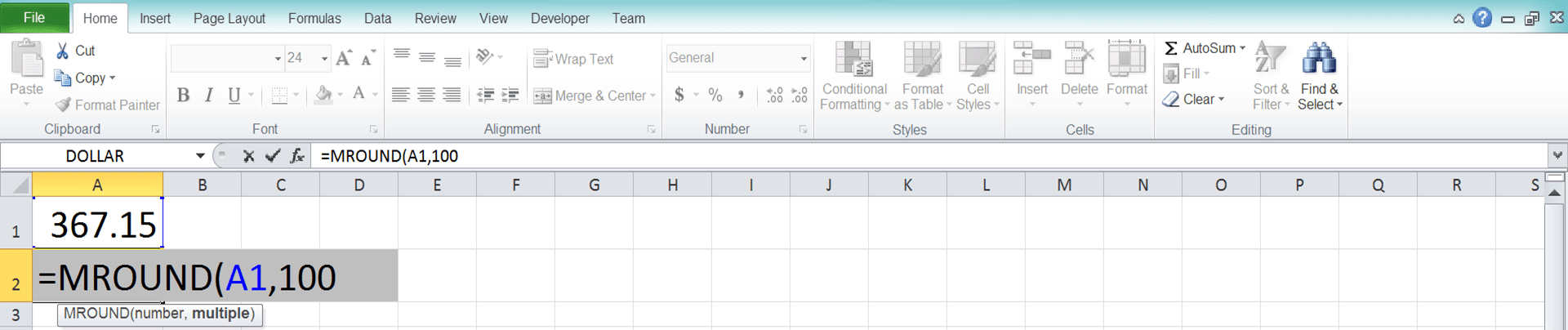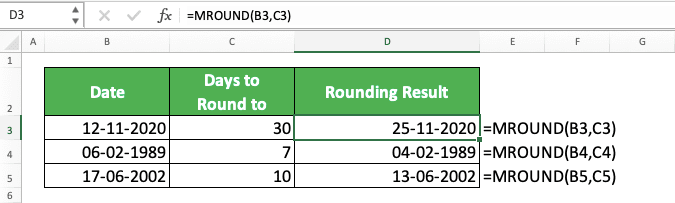How to Use the MROUND Excel Formula: Functions, Examples, and Writing Steps - Compute Expert

# How to Use the MROUND Excel Formula: Functions, Examples, and Writing Steps

Home >> Excel Tutorials from Compute Expert >> Excel Formulas List >> How to Use the MROUND Excel Formula: Functions, Examples, and Writing Steps

In this tutorial, you will learn how to use the MROUND excel formula completely.

When working with numbers in excel, we might sometimes want to round our number to a certain multiple. If we use MROUND to help us with the rounding process, we can get our rounding result easily and fast.

Want to know more about this MROUND formula and master the way to use it in excel? Read this tutorial until its last part!

Disclaimer: This post may contain affiliate links from which we earn commission from qualifying purchases/actions at no additional cost for you. Learn more

## What is the MROUND Formula in Excel?

MROUND is an excel formula that helps you to round your number to a certain multiple.

## MROUND Function in Excel

You can use MROUND to get the multiple rounding result of your number.

## MROUND Result

The MROUND result is the number that is the multiple rounding result of your number.

## Excel Version from Which We Can Start Using MROUND

You can use MROUND in excel since excel 2003.

## The Way to Write It and Its Inputs

Here is the general writing form of MROUND in excel.

= MROUND ( number_to_round , multiple )

And here is a bit explanation of the inputs you need to give when you write MROUND
• number_to_round = the number you want to round to a certain multiple
• multiple = the multiple you want to round your number to

## Example of Its Usage and Result

Here is an implementation example of MROUND in excel.As you can see in the example, it is quite easy to use MROUND in excel. Just input the number you want to round and its multiple reference, press enter, and you will immediately get your result!

MROUND can round your number up or down depending on which one is closer to the multiple.

## Writing Steps

After we have discussed the MROUND writing form, inputs, and implementation example, now let’s discuss its writing steps. As MROUND only needs two simple inputs, these writing steps should be easy to understand.

1. Type an equal sign ( = ) in the cell where you want to put your MROUND result2. Type MROUND (can be with large and small letters) and an open bracket sign after =3. Input the number you want to round to a certain multiple. Then, type comma sign ( , )4. Input the multiple you want to round your number into5. Type a close bracket sign6. Type Enter
7. Done!## MROUND Not Working/Gives an Error? Possible Reasons & Solutions

Your MROUND gives you a wrong result or an error? There are several things that can cause that to happen. The following will give you probably the most possible reasons and their solution.

• Reason: You input a positive number/multiple and a negative multiple/number to your MROUND
Solution: MROUND will give you an error if you input a number and a multiple with a different positive/negative affiliation. Change your inputs into all positive or all negative

• Reason: You input your number and multiple in the wrong order
Solution: If you identify this mistake, reverse the order of your inputs immediately

• Reason: You use MROUND to round decimals
Solution: Some people may mistake MROUND as an excel formula they can use to round decimals. However, MROUND rounds a number to a multiple instead. Use ROUND if you want to round the decimals in your number

Hope those points can make you get your rounding result right in excel!

## MROUND to Round Date

By using MROUND, you can also round your date data in excel. MROUND will round the date you input into it to a certain number of days multiple.

Here is the general writing form of MROUND when you use it to round date in excel.

= MROUND ( date_to_round , number_of_days_multiple )

And here is its implementation example.## MROUND to Round Time

You can also round your time data with MROUND. For this, you should input your multiple in the form of time too to get the result you want easier.

Here is the general writing form of MROUND when we use it to round time.

= MROUND ( time_to_round , time_multiple )

And here is its implementation example.## Exercise

After you understand how to use MROUND in excel completely, practice your understanding by doing the exercise below!

### Questions

1. Round the number in question 1 column to the nearest multiple of 100!
2. Round the number in question 2 column to the nearest multiple of 7!
3. Round the number in question 3 column to the nearest multiple of 250!

Date and time are also numbers in excel (their real form is a number. We just display them differently in comparison to standard numbers). That is why you can round them with MROUND too.

Related tutorials you should learn from:

Get updated excel info from Compute Expert by registering your email. It's free!